Animations

Producing animations are very simple. We have already met some examples using Manipulate which provides lower-level control over animations, but anything that produces can be turned into an animation by animating over a parameter. Here are some simple examples: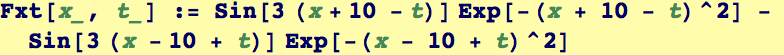Here is a simple plot at "time" = 0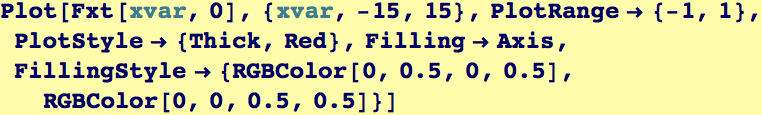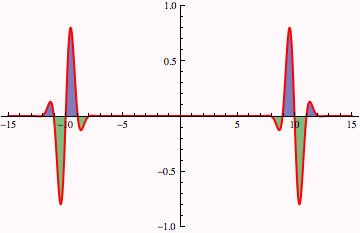To Animate :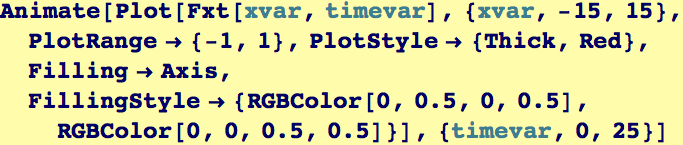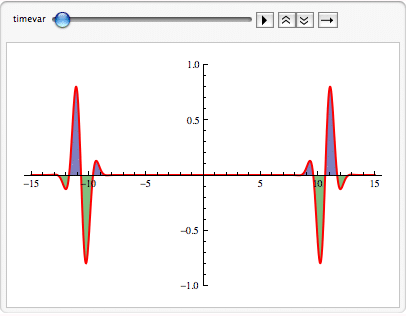This is the solution to the temperature evolution equation (the diffusion equation) for a square of length L initially at 500K embedded in a plate initially at 100K , κ is the themal diffusivity (units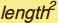/time).  We introduce a "normalized" time and space variables variable τ= κ t/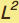and ξ = x/L and η=y/L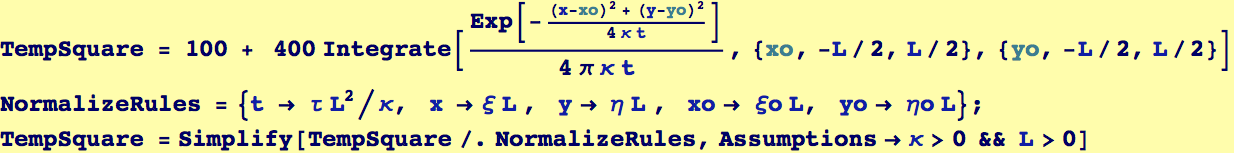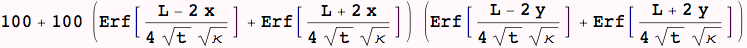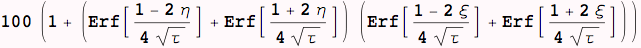We divide by 500 so that the temperatures should scale between zero and one, and then use ColorFunctionScaling->False so that the colors are consistent over time.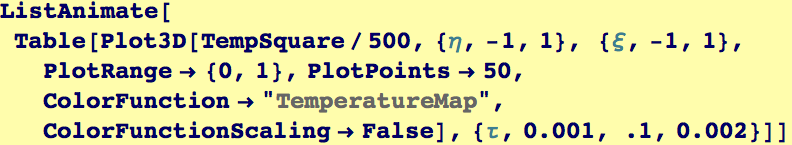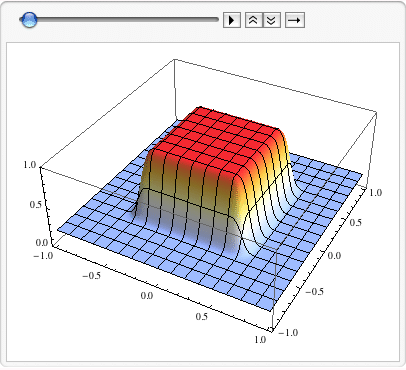Created by Wolfram Mathematica 6.0  (28 August 2007)